# K-Means聚类算法原理及Python实践

“聚类”（Clustering）试图将数据集中的样本划分为若干个不相交的子集，每个子集被称为一个“簇”或者“类”，英文名为Cluster。比如鸢尾花数据集（Iris Dataset）中有多个不同的子品种：Setosa、Versicolor、Virginica，不同品种的一些观测数据是具有明显差异的，我们希望根据这些观测数据将其进行聚类。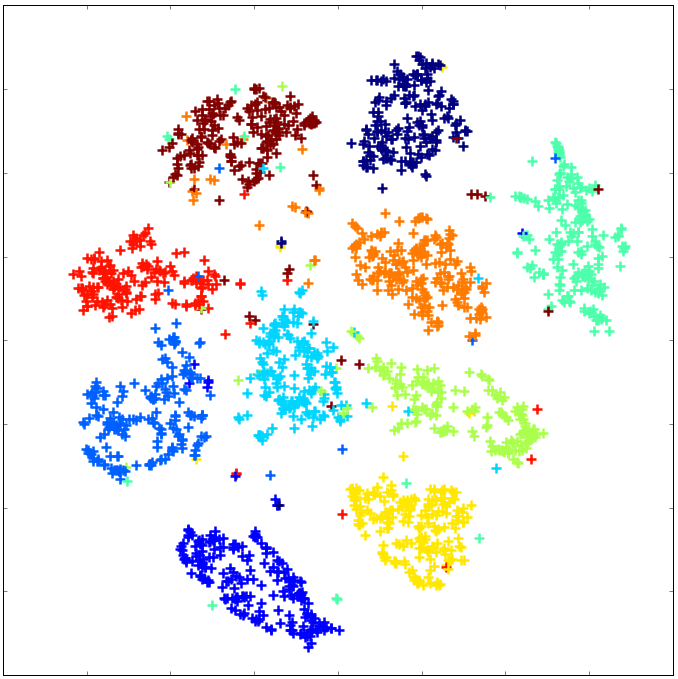不同鸢尾花的花萼长度和宽度不同

## K-Means算法​

K均值（K-Means）算法是最常用的聚类算法。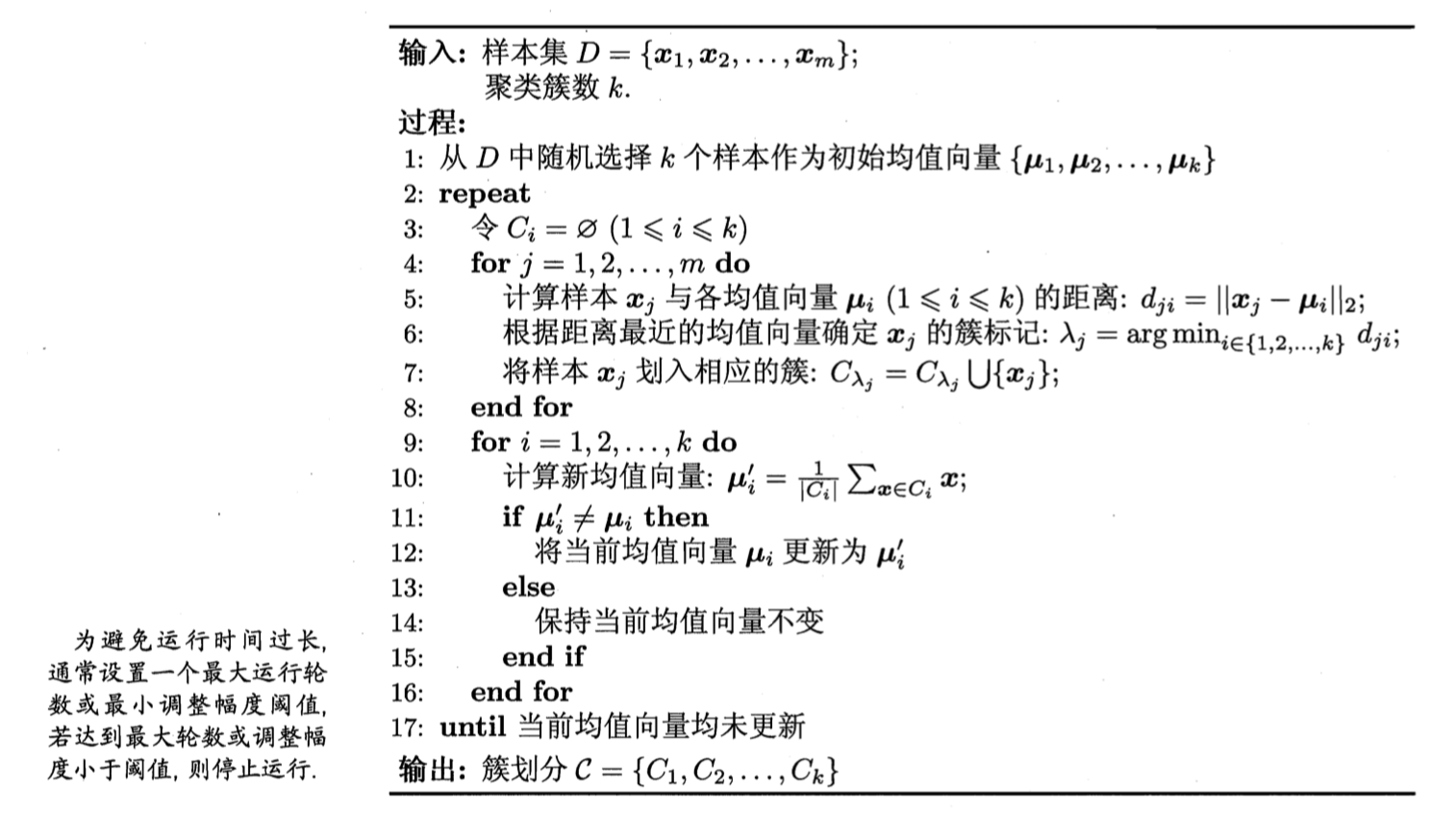K-Means算法的伪代码 来源：周志华《机器学习》

1. 在数据集D中随机选择K个点，这K个点表示K个簇的中心点，即伪代码第1行。
2. 计算数据集D中各个数据应该被分到哪些簇：具体而言，计算样本中所有点距离这K个中心点的距离，将某个样本x划分到距离其最近的中心点对应的簇上，如伪代码的4-8行。
3. 优化当前的聚类结构：对于刚刚生成的分类簇，重新计算簇的中心点，如伪代码的9-16行。
4. 重复前面两步，直到我们得到一个满意的结果。

K-Means算法是一种采用贪心思想的迭代算法。下图展示了从初始状态开始进行的4次迭代，每次迭代，簇的中心点和簇内数据点也在变化。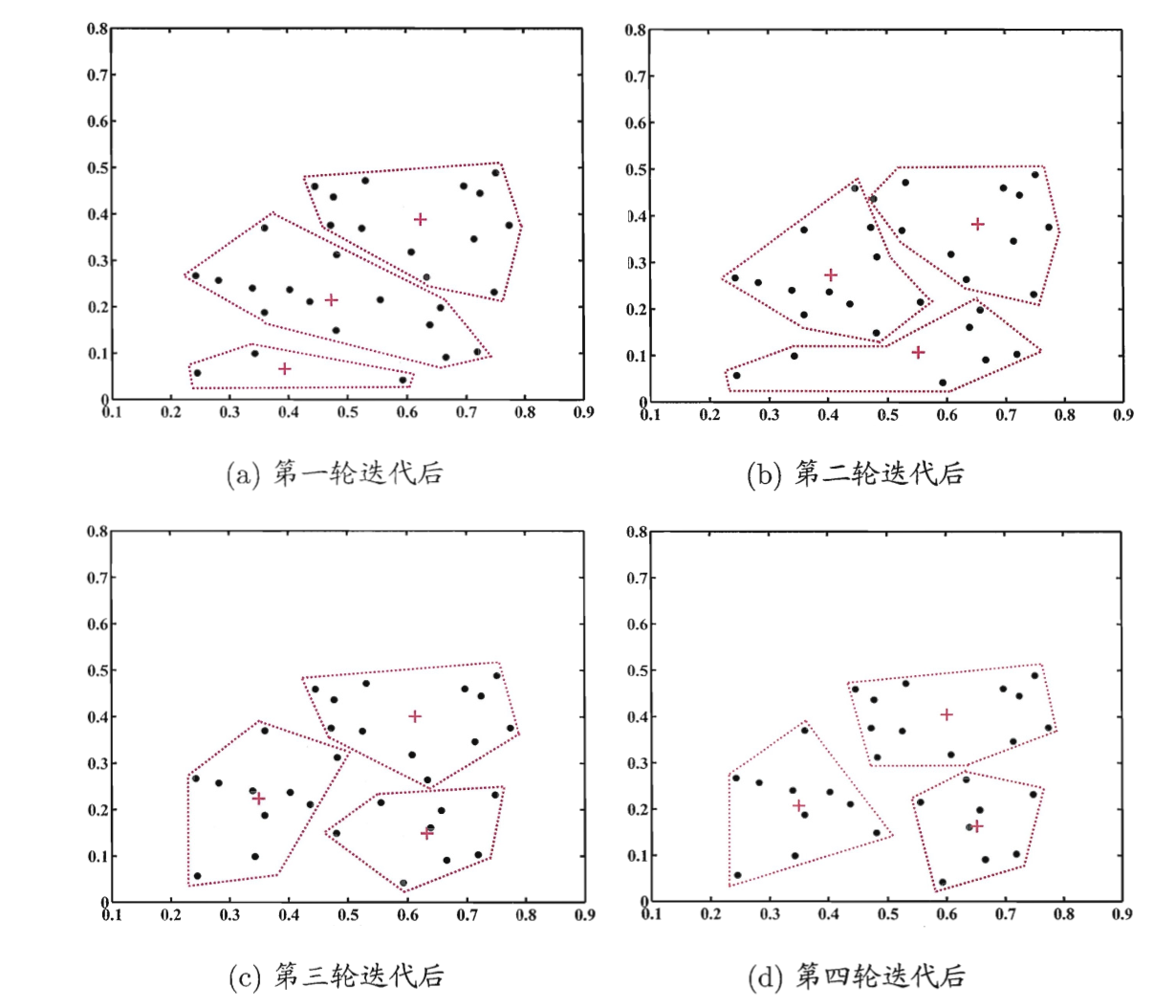将数据集分为3个簇，四轮迭代的结果，样本点为“·”，簇中心点为“+” 来源：周志华《机器学习》

## 使用scikit-learn对Iris数据集进行聚类​

Iris数据集共有3种类别的鸢尾花，每种50个样本。数据集提供了4个特征：花萼长度（Sepal Length）、花萼宽度（Sepal Width）、花瓣长度（Petal Length）和花瓣宽度（Petal Width）。

### 数据探索​

from sklearn import datasetsimport matplotlib.pyplot as pltimport pandas as pdfrom sklearn.cluster import KMeansiris = datasets.load_iris()### 对数据集进行探索# 打印数据集特征print("特征名：" + str(iris.feature_names))# 探索一个样本数据，每行数据4列，分别表示上述4种特征print("第一行样本：" + str(iris.data[:1]))# 第一个样本属于哪个品种，用数字代号表示print("第一行样本所属品种：" + str(iris.target[:1]))

特征名：['sepal length (cm)', 'sepal width (cm)', 'petal length (cm)', 'petal width (cm)']第一行样本：[[5.1 3.5 1.4 0.2]]第一行样本所属品种：

# 查看前两列数据，即花萼的长度和宽度X = iris.data[:, :2]y = iris.target# 将数据集中所有数据进行二维可视化展示plt.scatter(X[:,0], X[:,1], c=y, cmap='gist_rainbow')plt.xlabel('Spea1 Length', fontsize=12)plt.ylabel('Sepal Width', fontsize=12)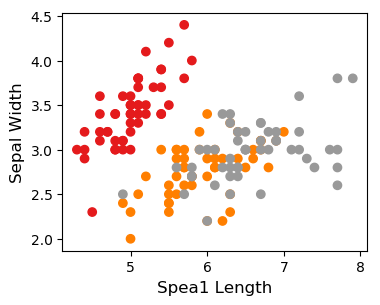花萼长度和宽度散点图可视化

# 为了更好理解数据集，使用PCA建模，观察3D模式下数据的分布fig = plt.figure(1, figsize=(8, 6))ax = Axes3D(fig, elev=-150, azim=110)X_reduced = PCA(n_components=3).fit_transform(iris.data)ax.scatter(X_reduced[:, 0], X_reduced[:, 1], X_reduced[:, 2], c=y,            cmap=plt.cm.Set1, edgecolor='k', s=40)ax.set_title("PCA建模")ax.set_xlabel("1st eigenvector")ax.w_xaxis.set_ticklabels([])ax.set_ylabel("2nd eigenvector")ax.w_yaxis.set_ticklabels([])ax.set_zlabel("3rd eigenvector")ax.w_zaxis.set_ticklabels([])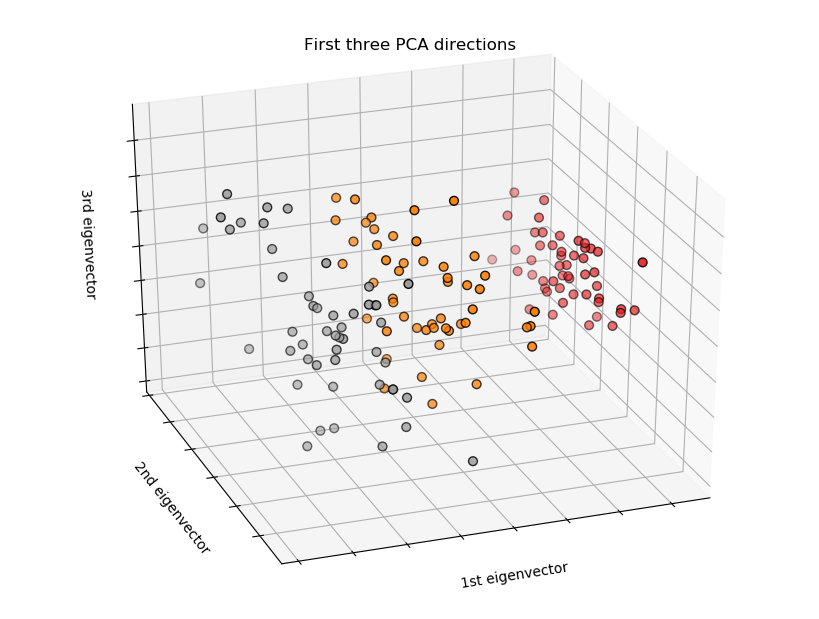PCA 3D数据分布

### 使用K-Means算法进行聚类分析​

### 对数据集使用K-Means进行聚类km = KMeans(n_clusters = 3)km.fit(X)# 打印聚类后各个簇的中心点centers = km.cluster_centers_print(centers)

[[5.006      3.428     ] [6.81276596 3.07446809] [5.77358491 2.69245283]]

# 比较聚类效果与真实品种之间的差异predicted_labels = km.labels_fig, axes = plt.subplots(1, 2, figsize=(16,8))axes.scatter(X[:, 0], X[:, 1], c=y, cmap=plt.cm.Set1,                 edgecolor='k', s=150)axes.scatter(X[:, 0], X[:, 1], c=predicted_labels, cmap=plt.cm.Set1,                edgecolor='k', s=150)axes.set_xlabel('Sepal length', fontsize=16)axes.set_ylabel('Sepal width', fontsize=16)axes.set_xlabel('Sepal length', fontsize=16)axes.set_ylabel('Sepal width', fontsize=16)axes.tick_params(direction='in', length=10, width=5, colors='k', labelsize=20)axes.tick_params(direction='in', length=10, width=5, colors='k', labelsize=20)axes.set_title('Actual', fontsize=18)axes.set_title('Predicted', fontsize=18)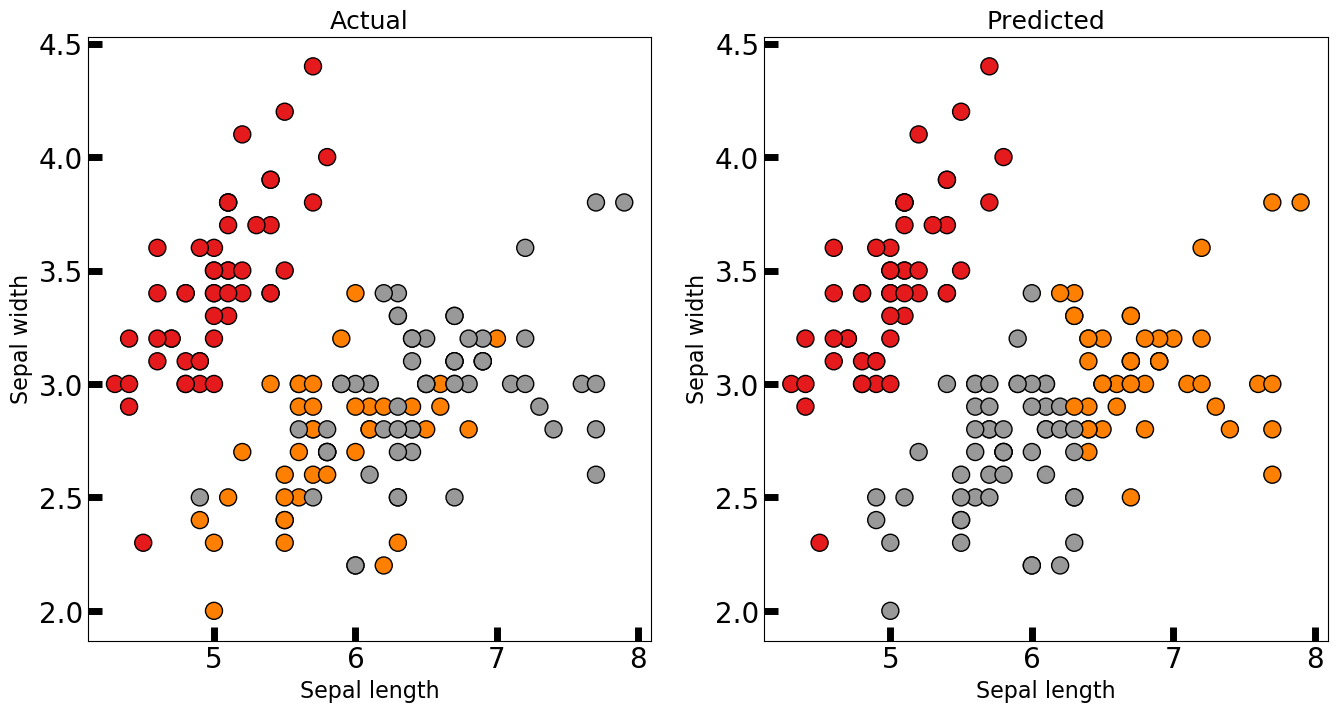K-Means聚类后，聚类结果和实际样本之间的差别图